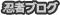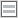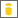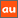# 0除算に関するアナウンスメントを公開しています

The purpose of this site is to publish announcements on the theory of division by zero

## Announcement 275: The division by zero $z/0=0$ and special relative theory of Einstein

Institute of Reproducing Kernels
Kawauchi-cho, 5-1648-16,
Kiryu 376-0041, Japan
E-mail: kbdmm360@yahoo.co.jp
January 11, 2016
Abstract: In this announcement, for its importance, we will state a fundamental result for special relative theory of Einstein from the division by zero $z/0=0$.

Introduction
By a natural extension of the fractions
\begin{equation}
\frac{b}{a} \tag{0.1}
\end{equation} for any complex numbers $a$ and $b$, the division by zero
\begin{equation}
\frac{b}{0}=0, \tag{0.2}
\end{equation} is clear and trivial. See $()$ for the recent results. See also the survey style announcements 179,185,237,246,247,250 and 252 of the Institute of Reproducing Kernels $(, ,, ,,,)$. The division by zero is not only mathematical problems, but also it will give great impacts to human beings and the idea on the universe. The Institute of Reproducing Kernels is presenting various; opinions in Announcements $($many in Japanese$)$ on the universe.
In this Announcement, for its importance, we will state a fundamental result for special relative theory of Einstein from the division by zero $z/0=0$. The contents were stated by Hiroshi Michiwaki in his memo dated on October 10, 2014 and we should state the results, more early.

1. Special relative theory of Einstein
Einstein's discovery of the equivalence of matter/mass and energy  in the year 1905 lies at the core of today's modern physics. According to Albert Einstein , the rest-mass $m_0$, a measure of the inertia of a $($quantum mechanical$)$ object is related to the relativistic mass $m_R$ by the equation, with relative velocity $v$ and the speed $c$ of light in vacuum,
\begin{equation}
m_0 = m_R \sqrt{1 - \frac{v^2}{c^2}}. \tag{1.1}
\end{equation} Therefore, we obtain, immediately
\begin{equation}
m_R^2= m_0^2 \left(1 - \frac{v^2}{c^2}\right)^{-1}. \tag{1.2}
\end{equation} Therefore, by the division by zero, we have the surprising result for $v = c$:
\begin{equation}
m_R = 0. \tag{1.3}
\end{equation} It seems that the modern physical common sense is then $m_R = + \infty$.

2. A conjecture by H. Michiwaki
As his simple result $(1.3)$ from the division by zero, Michiwaki stated his conjecture or interpretation for neutrino; neutrino are able to have small mass, because they are moved with near $c$ or $c$ velocity. Indeed, we assume that $m_0$ is the mass of neutrino at the stopped case. As the experiment, we know that the velocity of neutrino is near to $c$ or $c$. So he thought that neutrino will have small mass.
This result was realized positively by Takaaki Kajita by experiment and he got Novel Prize in 2015.
Furthermore, he referred to the very interesting interpretations of photon of energy and Doppler effect from the viewpoint of the division by zero in his memo.

Acknowledgements
This announcement was, of course,inspired by the paper  and for the very interesting relation with computer sciences and the division by zero, see.

References
 Barukcic J. P., and I. Barukcic, Anti Aristotle - The Division Of Zero By Zero,
ViXra.org $($Friday, June 5, 2015$)$ © Ilija Barukčić, Jever, Germany. All rights reserved. Friday, June 5, 2015 20:44:59.
 Bergstra, J. A., Hirshfeld Y., and Tucker, J. V.,
Meadows and the equational specification of division $($arXiv:0901.0823v1[math.RA] 7 Jan 2009$)$.
 Castro, L. P., and Saitoh, S. $($2013$)$. Fractional functions and their representations. Complex Anal. Oper. Theory 7, no. 4, 1049-1063.
 Einstein, A. $($1905$)$. Ist die Trägheit eines Körpers von seinem Energieinhalt abhängig?, Annalen der Physik, vol. 323, Issue 13, pp. 639-641,
 Einstein, A. $($1905$)$. Zur Elektrodynamik bewegter Körper, Annalen der Physik, vol. 322, Issue 10, pp. 891-921.
 Kuroda, M., Michiwaki, H., Saitoh, S., and Yamane, M. $($2014$)$. New meanings of the division by zero and interpretations on $100/0=0$ and on $0/0=0$,
Int. J. Appl. Math. Vol. 27, No 2, 191-198, DOI: 10.12732/ijam.v27i2.9.
 Michiwaki H., Saitoh S., and Yamada M. $($2015$)$. Reality of the division by zero $z/0=0$. IJAPM International J. of Applied Physics and Math. $($to appear$)$.
 Michiwaki, H., Saitoh, S., and Takagi, M. A new concept for the point at infinity and the division by zero $z/0=0$ $($manuscript$)$.
 Saitoh, S. $($2014$)$. Generalized inversions of Hadamard and tensor products for matrices, Advances in Linear Algebra & Matrix Theory. Vol.4 No.2 , 87-95. http://www.scirp.org/journal/ALAMT/
 Takahasi, S.-E. $($2014$)$. On the identities $100/0=0$ and $0/0=0$. $($note$)$
 Takahasi, S.-E., Tsukada, M., and Kobayashi, Y. $($2015$)$. Classification of continuous fractional binary operations on the real and complex fields. Tokyo Journal of Mathematics 8, no.2$($in press$)$.
 Division by zero is clear as z/0=0 and it is fundamental in mathematics. Announcement 179 $($2014.8.30$)$.
 The importance of the division by zero $z/0=0$. Announcement 185 $($2014.10.22$)$.
 A reality of the division by zero $z/0=0$ by geometrical optics. Announcement 237 $($2015.6.18$)$.
 An interpretation of the division by zero $1/0=0$ by the gradients of lines. Announcement 246 $($2015.9.17$)$.
 The gradient of y-axis is zero and $\tan (\pi/2) =0$ by the division by zero $1/0=0$. Announcement 247 $($2015.9.22$)$.
 What are numbers? - the Yamada field containing the division by zero $z/0=0$. Announcement 250 $($2015.10.20$)$.
 Circles and curvature - an interpretation by Mr. Hiroshi Michiwaki of the division by
zero $r/0 = 0$. Announcement 252 $($2015.11.1$)$.

PR

### コメント

お名前 Black LimeGreen SeaGreen Teal FireBrick Tomato IndianRed BurlyWood SlateGray DarkSlateBlue LightPink DeepPink DarkOrange Gold DimGray Silverプロフィール

ＨＮ：

(01/17)
(01/14)
(01/12)
(01/04)
(01/03)